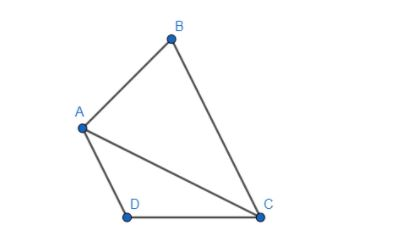QuestionAnswers

# If ABCD is a quadrilateral, then $\overline{AC}=\overline{AD}-\_\_\_\_\_$ .(a) $\overline{AD}$ (b) $\overline{BC}$ (c) $\overline{CD}$(d) $\overline{AC}$

Hint: As ABCD is a quadrilateral and ACD is a triangle which is also a polygon, we can use the rule of vector addition of a polygon to solve the above question. According to the rule the sum of the vectors forming all the sides of the polygon such that the tail of one vector coincides with the head of the other vector is zero. Also, use the identity that $\overline{DA}=-\overline{AD}$ .$\overline{DA}+\overline{CD}+\overline{AC}=0$
$\Rightarrow \overline{AC}=-\overline{DA}-\overline{CD}$
We also know that $\overline{DA}=-\overline{AD}$ .
$\overline{AC}=\overline{AD}-\overline{CD}$